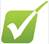Ideal gas equation and moles questions1)    If I have 40 moles of a gas at a pressure of 56000 Pa and a volume of 2 m3, what is the temperature?

2)    If I have an unknown quantity of gas at a pressure of 120000 Pa, a volume of 31 dm3, and a temperature of 360 k, how many moles of gas do I have?

3)    If I contain 3 moles of gas in a container with a volume of 60 dm3 and at a temperature of 400 K, what is the pressure inside the container?

4)    If I have 7.7 moles of gas at a pressure of 9 kPa and at a temperature of 56 0C, what is the volume of the container that the gas is in?

5)    An unknown gas weighs 34g and occupies 6.7 dm3 at 200 kPa and 245K. What is its relative molecular mass?

6)    What is the volume of 23g of Argon gas at 1 oC and a pressure of 200 kPa?

7)    Calculate the mass of a sample of carbon dioxide which occupies 20 dm3 at 27 oC and 100 kPa.

8)    Calculate the relative molecular mass of a gas if a 500 cm3 sample at 20 oC and 100 kPa has a mass of 0.66 g.